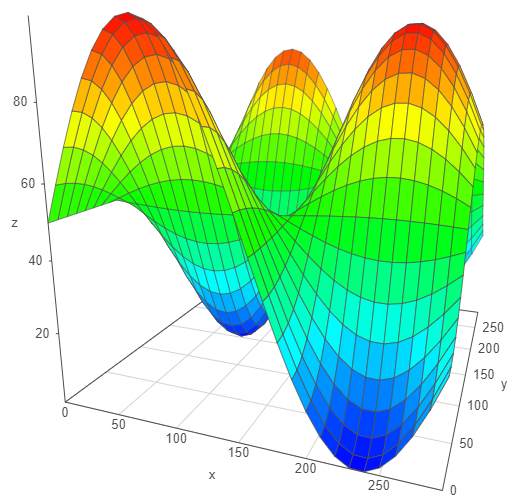# surface3d example color fixed to z axis values

I’m trying to set the surface color to something other than the z axis values but the surface color does not change.

The example from https://github.com/bokeh/bokeh/tree/master/examples/app/surface3d has the lines:

value = np.sin(xx/50) * np.cos(yy/50) * 50 + 50

``
source = ColumnDataSource(data=dict(x=xx, y=yy, z=value, color=value))

surface = Surface3d(x=“x”, y=“y”, z=“z”, color=“color”, data_source=source, width=600, height=600)

I’ve updated as follows:

value = np.sin(xx/50) * np.cos(yy/50) * 50 + 50

color = np.linspace(0, 1, value.shape)

source = ColumnDataSource(data=dict(x=xx, y=yy, z=value, color=color))

surface = Surface3d(x=“x”, y=“y”, z=“z”, color=“color”, data_source=source, width=600, height=600)

``

But the image remains the same as the attached png.

Version Info···

Python version 2.7.13

Anaconda2 Distribution 4.4.0 Community Edition

Bokeh version 0.12.5

nodejs version 9.11.0

Windows 8.1 Pro (Build 9600)

Chrome Version 65.0.3325.181 (Official Build) (64-bit)

Hi,

As I mentioned in https://github.com/bokeh/bokeh/issues/7814#issuecomment-382852349 this is a limitation of the underlying Graph3d library, so there is nothing to do (except remove the extraneous color property from the example altogether). This example is merely meant to be illustrative of the techniques of wrapping an external JS lib, not necessarily complete or very useful on its own.

Thanks,

Bryan

···

On Apr 19, 2018, at 12:00, [email protected] wrote:

I'm trying to set the surface color to something other than the z axis values but the surface color does not change.

The example from https://github.com/bokeh/bokeh/tree/master/examples/app/surface3d has the lines:
value = np.sin(xx/50) * np.cos(yy/50) * 50 + 50
source = ColumnDataSource(data=dict(x=xx, y=yy, z=value, color=value))
surface = Surface3d(x="x", y="y", z="z", color="color", data_source=source, width=600, height=600)

I've updated as follows:
value = np.sin(xx/50) * np.cos(yy/50) * 50 + 50
color = np.linspace(0, 1, value.shape)
source = ColumnDataSource(data=dict(x=xx, y=yy, z=value, color=color))
surface = Surface3d(x="x", y="y", z="z", color="color", data_source=source, width=600, height=600)

But the image remains the same as the attached png.

Version Info
----------------------------------------------------------------------------------------
Python version 2.7.13
Anaconda2 Distribution 4.4.0 Community Edition
Bokeh version 0.12.5
nodejs version 9.11.0
Windows 8.1 Pro (Build 9600)
Chrome Version 65.0.3325.181 (Official Build) (64-bit)

--
You received this message because you are subscribed to the Google Groups "Bokeh Discussion - Public" group.
To unsubscribe from this group and stop receiving emails from it, send an email to [email protected].
To post to this group, send email to [email protected].
To view this discussion on the web visit https://groups.google.com/a/continuum.io/d/msgid/bokeh/673729e6-6765-4d1d-b917-9b70f4e2fb6a%40continuum.io.